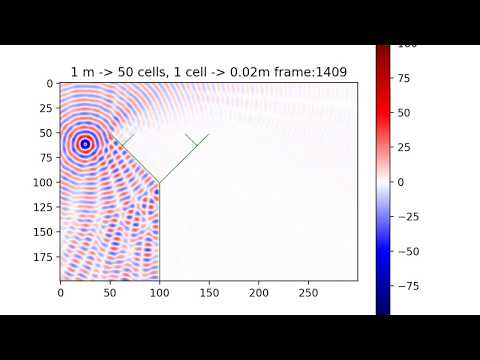# Data visualization in Python, Part 1: Cellular Automata

Python wasn’t part of my university curriculum and by the third year I knew only the basics. I've heard about this language and how easy it was to program in it with the help of libraries that do wonders in just a few lines.

I decided to learn Python. And what better way to do that than by working on a practical example? I chose to try Python’s strength in data visualization in a project that would simulate cellular automata.

A cellular automaton can be represented as a two-dimensional array of values ​​that change according to established rules, on the basis of values in neighboring cells. They are applicable in various simulations such as sea surface, road traffic, and fire spread.

Have a look here to see some examples:

In my project, I wanted to simulate the propagation of a sound wave based on this research study:

Komatsuzaki T., Iwata Y., Morishita S. (2012) Modeling Sound Wave Propagation around Sound Barriers Using Cellular Automata. In: Sirakoulis G.C., Bandini S. (eds) Cellular Automata. ACRI 2012. Lecture Notes in Computer Science, vol. 7495. Springer, Berlin, Heidelberg.

The principle of operation was very simple. Each cell contains information about the current pressure and air flow rate from neighboring cells. In each step of the simulation, we calculate the new pressure value based on the previous values.

Naturally, you might immediately jump into the conclusion that if we need to present 2D data, we also need a chart library - and that’s where Matplotlib helps us. Looking at numerous examples, we can see that drawing a graph takes just a few lines of code.

We simulate a space of 6x4m which gives us 60,000 cells.

The entire code is available under this link: https://github.com/Alexander3/wave-propagation,  note that commit names correspond to the next steps.

We need to display an array of numbers in the range [-100, 100] - what would we expect from the library?

• We want the range of values ​​to be mapped to a range of colors with linear interpolation,

• We want the graph itself to be interpolated as well,

• We want to see a bar showing what color corresponds to what value.

``````pressure = [[sin(x + y) for x in range(size_x)] for y in range(size_y)] min_presure = -1 max_pressure = 1

ca_plot = plt.imshow(pressure, interpolation='bilinear', vmin=min_presure, vmax=max_pressure) plt.colorbar(ca_plot) plt.show()
``````

Nice! 20 lines in total and we have plot with gui that allows us to zoom, pan and save what we see.

Step 1: We can display 2d data so let's deal with the simulation. The first step is calculating the outflow rate for all cells, knowing pressure difference.

``````for i in range(size_y): for j in range(size_x): cell_pressure = P[i][j] V[i][j] = V[i][j] + cell_pressure - P[i - 1][j] if i > 0 else cell_pressure V[i][j] = V[i][j] + cell_pressure - P[i][j + 1] if j < size_x - 1 else cell_pressure V[i][j] = V[i][j] + cell_pressure - P[i + 1][j] if i < size_y - 1 else cell_pressure V[i][j] = V[i][j] + cell_pressure - P[i][j - 1] if j > 0 else cell_pressure

``````

We then simulate the flow itself - that is, we update the pressure taking in account outflow velocities. A little more code and we can see the result:

``````for i in range(size_y): for j in range(size_x): self.pressure[i][j] -= 0.5 * damping * sum(self._velocities[i][j])

# main.py simulation = Simulation()

for i in range(50): simulation.step() ca_plot = plt.imshow(simulation.pressure, interpolation='bilinear', vmin=min_presure, vmax=max_pressure) plt.colorbar(ca_plot) plt.show()
``````

You should be able to see something like that. Ok, we get a picture with the result of our simulation. But for a better illustration of the problem, an animation of the wave propagation would be useful. `matplotlib.animation.FuncAnimation` serves this purpose.You should be able to see something like this

Step 2: A few extra lines of code…

``````def animation_func(i): simulation.step() ca_plot.set_data(simulation.pressure) return ca_plot

animation = FuncAnimation(figure, animation_func, interval=1)

``````

After running the code, we can  see an animation. It’s quite slow so we can use numpy.array and start optimizing the code (actually, when I tried that once, the time of a single step increased from 91.5 ms to 279 ms). We can also use CUDA here. But what we want is to generate a film rather than than display real-time animation, so let's leave optimization for the next time.

Step 3: Maximize the window, add a description, and change the colors - at this point, I recommend reviewing these color maps.

``````ca_plot = plt.imshow(simulation.pressure, cmap='seismic', interpolation='bilinear', vmin=min_presure, vmax=max_pressure)

mng = plt.get_current_fig_manager() mng.window.showMaximized() plt.title(f"1 m -> {scale} cells, 1 cell -> {1 / scale}m")

``````

Step 4: Let’s add a soundproof wall. I use another array to hold it’s location. In velocities calculation we just have to skip these cells.

``````if wall[i][j] == 1: V[i][j] = V[i][j] = V[i][j] = V[i][j] = 0.0 continue

``````Final result

Step 5: Video recording requires installation of ffmpeg.

``````writer = FFMpegWriter(fps=30) frames = 100 with writer.saving(figure, "writer_test.mp4", 200): for i in range(frames): animation_func(i) writer.grab_frame() print(f'\\rframe: {i}/{frames}', end='')

``````

You can see the result here:## What’s next?

I was quite pleased with the final result of my project. Plans for the future? It would be great to draw walls in graphics editor and then load them to our simulation.

When researching the problem, I noticed that a group of developers tried to do something similar, but using Java. Their code was 1,500 lines long - mine only 120 lines, all thanks to Python’s incredible conciseness and Matplotlib magic.